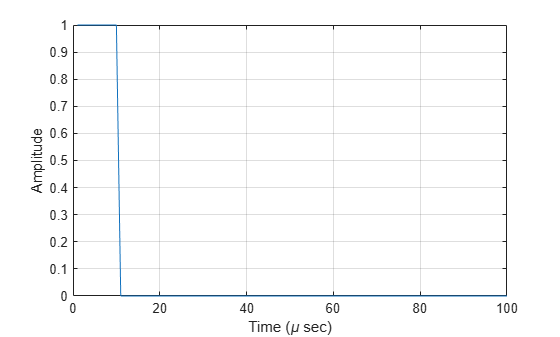Documentation

# step

System object: phased.RectangularWaveform
Package: phased

Samples of rectangular pulse waveform

## Syntax

Y = step(sRFM) Y = step(sRFM,prfidx) [Y,PRF] = step(___) 

## Description

### Note

Starting in R2016b, instead of using the step method to perform the operation defined by the System object™, you can call the object with arguments, as if it were a function. For example, y = step(obj,x) and y = obj(x) perform equivalent operations. When the only argument to the step method is the System object itself, replace y = step(obj) by y = obj().

Y = step(sRFM) returns samples of a rectangular pulse in the column vector Y.

Y = step(sRFM,prfidx), uses the prfidx index to select the PRF from the predefined vector of values specified by the PRF property. This syntax applies when you set the PRFSelectionInputPort property to true.

[Y,PRF] = step(___) also returns the current pulse repetition frequency, PRF. To enable this syntax, set the PRFOutputPort property to true and set the OutputFormat property to 'Pulses'.

.

### Note

The object performs an initialization the first time the object is executed. This initialization locks nontunable properties (MATLAB) and input specifications, such as dimensions, complexity, and data type of the input data. If you change a nontunable property or an input specification, the System object issues an error. To change nontunable properties or inputs, you must first call the release method to unlock the object.

## Examples

expand all

Construct a 10 microseconds rectangular pulse with a pulse repetition interval of 100 microseconds.

Pulsewidth = 10e-6; PRI = 100e-6; sRFM = phased.RectangularWaveform('PulseWidth',Pulsewidth,... 'OutputFormat','Pulses','NumPulses',1,... 'SampleRate',1e6,'PRF',1/PRI); wav = step(sRFM); plot(wav) xlabel('Time (\mu sec)') ylabel('Amplitude') gridConstruct rectangular waveforms with two pulses each. Set the sample rate to 1 MHz, a pulse width of 50 microseconds, and a duty cycle of 20%. Vary the pulse repetition frequency.

Set the sample rate and PRF. The ratio of sample rate to PRF must be an integer.

fs = 1e6; PRF = [10000,25000]; waveform = phased.RectangularWaveform('OutputFormat','Pulses','SampleRate',fs,... 'DurationSpecification','Duty Cycle','DutyCycle',.2,... 'PRF',PRF,'NumPulses',2,'PRFSelectionInputPort',true);

Obtain and plot the rectangular waveforms. For the first call to the step method, set the PRF to 10kHz using the PRF index. For the next call, set the PRF to 25 kHz. For the final call, set the PRF to 10kHz.

wav = []; wav1 = waveform(1); wav = [wav; wav1]; wav1 = waveform(2); wav = [wav; wav1]; wav1 = waveform(1); wav = [wav; wav1]; nsamps = size(wav,1); t = [0:(nsamps-1)]/waveform.SampleRate; plot(t*1e6,real(wav)) xlabel('Time (\mu sec)') ylabel('Amplitude') grid